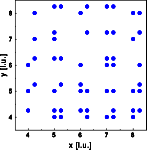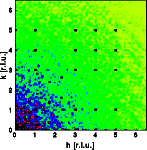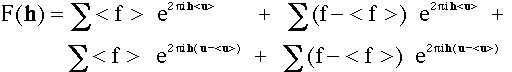Interactive Tutorial about Diffraction Diffuse scattering: Occupational disorder IDiffuse scattering Thermal I Thermal II Occupational I Occupational II Longitudinal waves Transversal waves Short range order Stacking faults Interactive examples Displacement waves Short range order Stacking faults Goto Contents The third example shows the effect of replacement disorder. We are using the identical crystal structure as in examples 1 and 2. The crystal consists of square molecules in a primitive cubic lattice. 50% of all atoms are replaced independently and at random by a vacancy. The left image shows a part of the crystal, 30*30 unit cells were used for the calculation. The right image shows one quadrant of reciprocal space.The Bragg reflections are shown as black dots, most of them are overexposed. Diffuse scattering results that is essentially monotonous in reciprocal space. Note that now the diffuse scattering is more intense at shorter reciprocal vectors h a typical phenomenon of replacement disorder. Lets look again at the formula from the first example:where < f > and < u > are the average form factor and the average position of the atom respectively. The sum is over all atoms in the crystal and f and u are the scattering factors of the current atom. The first sum gives the Bragg reflections. Now u - < u > is zero for all atoms. The term (f - < f > ) is largest near the center of reciprocal space and diminishes with the diminishing value of < f >. Note that this relationship holds only for X-ray diffraction! Since the scattering length f for neutron scattering is independent of the absolute value of h, the random replacement diffuse neutron scattering is independent of the location in reciprocal space. © Th. Proffen and R.B. Neder, 2003